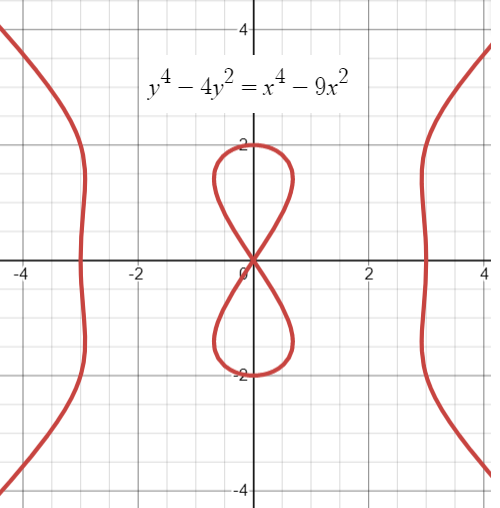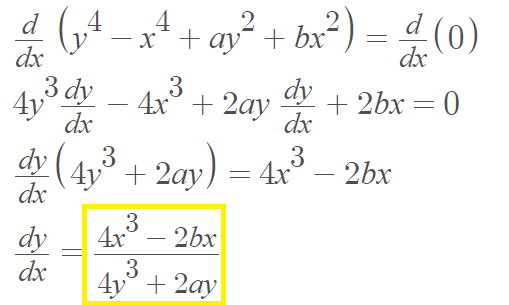# Devil’s Curve

## What is The Devil’s Curve?The Devil’s curve is the locus of the equation
y4 – x4 + ay2 + bx2 = 0.
The constant a is a linear distortion of the curve. When b = 25/24, the curve is called the electric motor curve .

The polar equation is r = √[25 – 24 tan2(θ))].

There is one double point at (0, 0).

The curve is symmetric about the y-axis and the x-axis.

## Slope of the Tangent Line for the Devil’s Curve

The slope of the tangent line for any point (x, y) on the curve can be found with implicit differentiation:## History of the Devil’s Curve

The Devil’s Curve was first studied by Gabriel Cramer (of Cramer’s rule fame) in 1750 in Introduction a l’Analyse des lignes courbes (p.19). It appears in the 1858 edition of Nouvelles Annales de Mathématiques..

The curve is called the “Devil’s curve” because the central leminiscate shape of the curve resembles the top used in the game of diabolo or la curbe du diable; the outer edges of the curve look like the sticks. The question “Can one furnish a reason for the peculiar name of the “devil’s curve”…” was posed more than a century ago in an an 1895 Mathematical of Association monthly journal. The answer from Professor J.F.W. Scheffer (p.96) was that while the genesis and history is “obscure,” Cramer may have given it the French name of the toy “la courbe du diable,” since the curve clearly characterizes the outline and symmetry of the “Devil on two sticks”.

## References

Graph created with Desmos.
 Fifty Famous Curves, Lots of Calculus Questions, And a Few Answers.
 Reid, B. & Marshall, B. The Devil’s Curve. Retrieved December 29, 2021 from: https://mse.redwoods.edu/darnold/math50c/CalcProj/Fall08/reidmarshall/TheDevilsCurve.ppt
 Double Top for Games.

CITE THIS AS:
Stephanie Glen. "Devil’s Curve" From StatisticsHowTo.com: Elementary Statistics for the rest of us! https://www.statisticshowto.com/devils-curve/